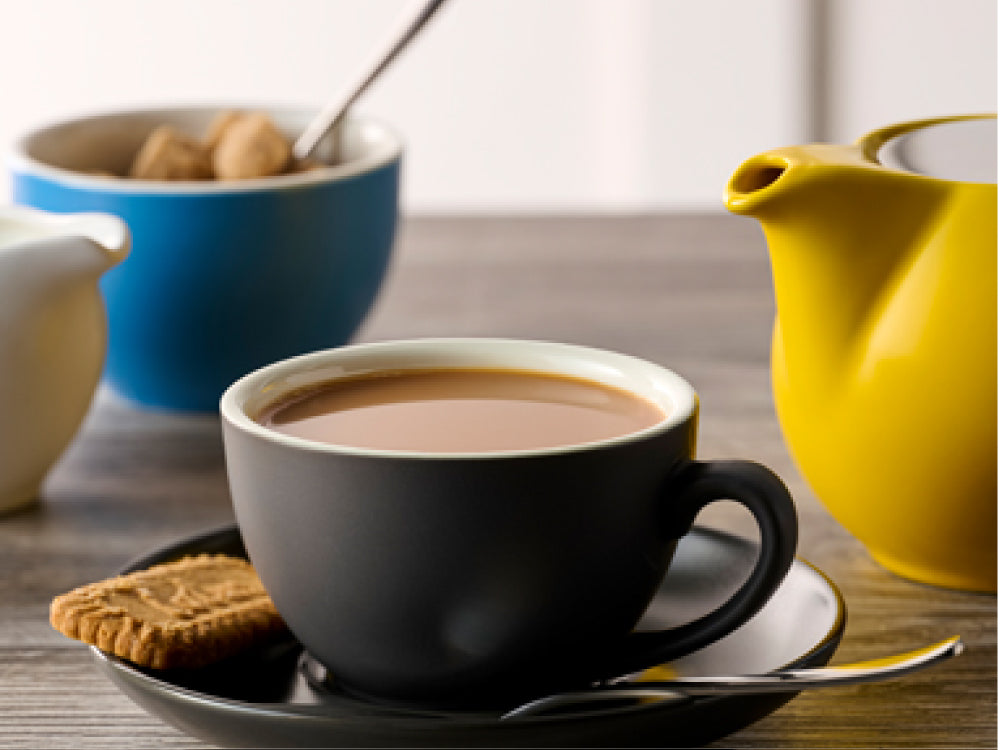Shopping Cart

### Refine

View all

#### Price# Coffee Bar£5.76 each (ex VAT)£2.24 each (ex VAT)£1.21 each (ex VAT)£1.40 each (ex VAT)£1.02 each (ex VAT)£2.57 each (ex VAT)£1.64 each (ex VAT)£2.84 each (ex VAT)£4.69 each (ex VAT)£3.23 each (ex VAT)£3.14 each (ex VAT)£2.20 each (ex VAT)£5.54 each (ex VAT)£4.06 each (ex VAT)£6.12 each (ex VAT)£5.70 each (ex VAT)£5.93 each (ex VAT)£6.64 each (ex VAT)£4.83 each (ex VAT)£2.96 each (ex VAT)£2.07 each (ex VAT)£2.99 each (ex VAT)£4.27 each (ex VAT)£3.54 each (ex VAT)£3.83 each (ex VAT)£11.25 each (ex VAT)£15.03 each (ex VAT)£2.74 each (ex VAT)£5.12 each (ex VAT)£3.76 each (ex VAT)£3.76 each (ex VAT)£5.12 each (ex VAT)£5.28 each (ex VAT)£5.28 each (ex VAT)£34.54 each (ex VAT)£19.78 each (ex VAT)£24.10 each (ex VAT)£12.32 each (ex VAT)£13.42 each (ex VAT)£38.58 each (ex VAT)£18.74 each (ex VAT)£22.20 each (ex VAT)£3.26 each (ex VAT)£3.26 each (ex VAT)£8.79 each (ex VAT)£10.94 each (ex VAT)£8.02 each (ex VAT)£7.70 each (ex VAT)